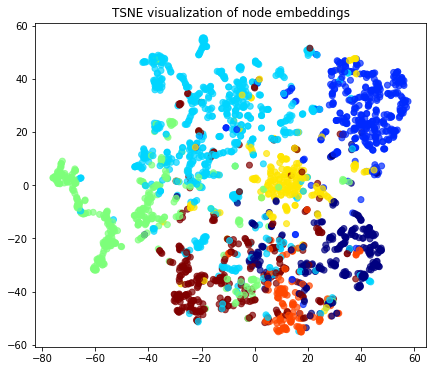# Node representation learning with Node2Vec¶

An example of implementing the Node2Vec representation learning algorithm using components from the stellargraph and gensim libraries.

References

 Node2Vec: Scalable Feature Learning for Networks. A. Grover, J. Leskovec. ACM SIGKDD International Conference on Knowledge Discovery and Data Mining (KDD), 2016. (link)

 Distributed representations of words and phrases and their compositionality. T. Mikolov, I. Sutskever, K. Chen, G. S. Corrado, and J. Dean. In Advances in Neural Information Processing Systems (NIPS), pp. 3111-3119, 2013. (link)

 Gensim: Topic modelling for humans. (link)

:

import matplotlib.pyplot as plt
from sklearn.manifold import TSNE
from sklearn.decomposition import PCA
import os
import networkx as nx
import numpy as np
import pandas as pd
from stellargraph import datasets
from IPython.display import display, HTML

%matplotlib inline


## Dataset¶

For clarity, we use only the largest connected component, ignoring isolated nodes and subgraphs; having these in the data does not prevent the algorithm from running and producing valid results.

:

dataset = datasets.Cora()
display(HTML(dataset.description))

The Cora dataset consists of 2708 scientific publications classified into one of seven classes. The citation network consists of 5429 links. Each publication in the dataset is described by a 0/1-valued word vector indicating the absence/presence of the corresponding word from the dictionary. The dictionary consists of 1433 unique words.
:

print(G.info())

StellarGraph: Undirected multigraph
Nodes: 2485, Edges: 5209

Node types:
paper: 
Edge types: paper-cites->paper

Edge types:
paper-cites->paper: 


## The Node2Vec algorithm¶

The Node2Vec algorithm introduced in  is a 2-step representation learning algorithm. The two steps are:

1. Use second-order random walks to generate sentences from a graph. A sentence is a list of node ids. The set of all sentences makes a corpus.

2. The corpus is then used to learn an embedding vector for each node in the graph. Each node id is considered a unique word/token in a dictionary that has size equal to the number of nodes in the graph. The Word2Vec algorithm  is used for calculating the embedding vectors.

## Corpus generation using random walks¶

The stellargraph library provides an implementation for second-order random walks as required by Node2Vec. The random walks have fixed maximum length and are controlled by two parameters p and q. See  for a detailed description of these parameters.

We are going to start 10 random walks from each node in the graph with a length up to 100. We set parameter p to 0.5 (which encourages backward steps) and q to 2.0 (which discourages distant steps); the net result is that walks should remain in the local vicinity of the starting nodes.

:

from stellargraph.data import BiasedRandomWalk

rw = BiasedRandomWalk(G)

walks = rw.run(
nodes=list(G.nodes()),  # root nodes
length=100,  # maximum length of a random walk
n=10,  # number of random walks per root node
p=0.5,  # Defines (unormalised) probability, 1/p, of returning to source node
q=2.0,  # Defines (unormalised) probability, 1/q, for moving away from source node
)
print("Number of random walks: {}".format(len(walks)))

Number of random walks: 24850


## Representation Learning using Word2Vec¶

We use the Word2Vec  implementation in the free Python library gensim , to learn representations for each node in the graph. It works with str tokens, but the graph has integer IDs, so they’re converted to str here.

We set the dimensionality of the learned embedding vectors to 128 as in .

:

from gensim.models import Word2Vec

str_walks = [[str(n) for n in walk] for walk in walks]
model = Word2Vec(str_walks, size=128, window=5, min_count=0, sg=1, workers=2, iter=1)

:

# The embedding vectors can be retrieved from model.wv using the node ID.
model.wv["19231"].shape

:

(128,)


## Visualise Node Embeddings¶

We retrieve the Word2Vec node embeddings that are 128-dimensional vectors and then we project them down to 2 dimensions using the t-SNE algorithm.

:

# Retrieve node embeddings and corresponding subjects
node_ids = model.wv.index2word  # list of node IDs
node_embeddings = (
model.wv.vectors
)  # numpy.ndarray of size number of nodes times embeddings dimensionality
node_targets = node_subjects.loc[[int(node_id) for node_id in node_ids]]


Transform the embeddings to 2d space for visualisation

:

transform = TSNE  # PCA

trans = transform(n_components=2)
node_embeddings_2d = trans.fit_transform(node_embeddings)

:

# draw the embedding points, coloring them by the target label (paper subject)
alpha = 0.7
label_map = {l: i for i, l in enumerate(np.unique(node_targets))}
node_colours = [label_map[target] for target in node_targets]

plt.figure(figsize=(7, 7))
plt.axes().set(aspect="equal")
plt.scatter(
node_embeddings_2d[:, 0],
node_embeddings_2d[:, 1],
c=node_colours,
cmap="jet",
alpha=alpha,
)
plt.title("{} visualization of node embeddings".format(transform.__name__))
plt.show()